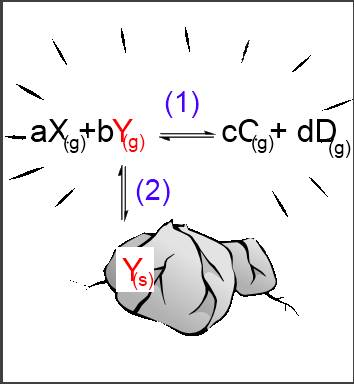Search:

# The heterogeneous equilibrium

## Definition

A heterogeneous equilibrium is a chemical equilibrium between species present in different phases. At least one of the species must have a constant concentration in one of its phases.

## The law of heterogeneous equilibrium

Let's suppose that reagent $Y$ occurs in the solid phase, the reagent $X$ and the products $C$ and $D$ in a gaseous phase: $aX_{(g)}+b$ $Y_{(s)}$$\leftrightarrows cC_{(g)} + dD_{(g)} (1) The reaction is only possible if Y passes first from the solid phase into the gaseous phase: Y_{(s)}$$\leftrightarrows$$Y_{(g)} (2) Now we have a homogeneous equilibrium (1) as well as a heterogeneous one (2):Let's apply the laws of mass action to these two equilibria: (1): \frac{[C_{(g)}]^c\cdot[D_{(g)}]^d}{[X_{(g)}]^a\cdot[Y_{(g)}]^b} = K_c (2): [Y_{(g)}] = K_{sat} (2) into (1) : \frac{[C_{(g)}]^c\cdot[D_{(g)}]^d}{[X_{(g)}]^a\cdot K_{sat}^b} = K_c \frac{[C_{(g)}]^c\cdot[D_{(g)}]^d}{[X_{(g)}]^a} = K_c\cdot K_{sat}^b K_c\cdot K_{sat}^b is a new constant K, so that: In case of an heterogeneous equilibrium aX_{(g)} + b Y_{(s)}$$ \leftrightarrows$ $cC_{(g)}$ $+$ $dD_{(g)}$, the following applies: $\frac{[C_{(g)}]^c\cdot[D_{(g)}]^d}{[X_{(g)}]^a}$ $=$ $K$ In the law of mass action, one leaves out the species present in the phase where its concentration is constant!